# Partially ordered group

(diff) ← Older revision | Latest revision (diff) | Newer revision → (diff)

A groupon which a partial order relationis given such that for all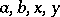inthe inequality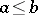implies.

The set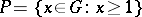in a partially ordered group is called the positive cone, or the integral part, ofand satisfies the properties: 1)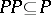; 2)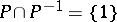; and 3)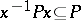for all. Any subsetofthat satisfies the conditions 1)–3) induces a partial order on(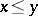if and only if) for whichis the positive cone.

Examples of partially ordered groups. The additive group of real numbers with the usual order relation; the group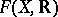of functions from an arbitrary setinto, with the operation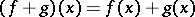and order relationiffor all; the group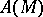of all automorphisms of a totally ordered setwith respect to composition of functions, and with order relation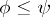iffor all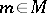, where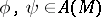.

The basic concepts of the theory of partially ordered groups are those of an order homomorphism (cf. Ordered group), a convex subgroup, and Cartesian and lexicographic products.

Important classes of partially ordered groups are totally ordered groups and lattice-ordered groups (cf. Totally ordered group; Lattice-ordered group).

How to Cite This Entry:
Partially ordered group. Encyclopedia of Mathematics. URL: http://encyclopediaofmath.org/index.php?title=Partially_ordered_group&oldid=13204
This article was adapted from an original article by V.M. Kopytov (originator), which appeared in Encyclopedia of Mathematics - ISBN 1402006098. See original article